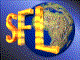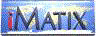| iMatix home page | << | < | > | >>SFLVersion 2.11

### http_multipart_decode

```#include "sflhttp.h"
DESCR *
http_multipart_decode (const char *mime_file, const char *store_path,
const char *local_format)
```

#### Synopsis

Parses a multipart-encoded file (as received by a web server as POST data) and returns a HTTP-encoded string containing the field data, in the format: "name=value&name=value&name=value...". For each field that refers to an uploaded file (INPUT field with type FILE), creates a temporary file holding the data. The name of this temporary file is put into a generated variable, whose name is built by using the local format string (ex: '%s_tmp'). The actual uploaded file is stored in a temporary file whose name is generated by the SFL get tmp file name() function. So, data for a file upload field called "doc" will be stored in a temporary file called (eg) "temp1234.tmp", and a field "doc_tmp" will be added, with the value "temp1234.tmp". The HTTP-encoded string is returned as a DESCR block, which you can decode using http query2strt(), passing the descriptor data. You must free the descriptor using mem_free() when you're finished with it.

#### Source Code - (sflhttp.c)

```{
FILE
*f_source,
*f_tmp = NULL;
char
*tmp_name = NULL,
*p_data,
*p_next,
*buffer;
int
offset,
static char
separator [80 + 1];
static int
sep_size;
SYMTAB
*table,
qbyte
tmp_index = 1;
DESCR
*descr = NULL;

ASSERT (local_format);

if (strstr (local_format, "%s") == NULL)
return (NULL);

buffer = mem_alloc (MULTI_BUFFER_SIZE + REST_BUFFER_SIZE + 1);
if (buffer == NULL)
return (NULL);

table = sym create table ();
if (table == NULL)
{
mem_free (buffer);
return (NULL);
}

header_tab = sym create table ();
{
mem_free (buffer);
sym delete table (table);
return (NULL);
}

f_source = fopen (mime_file, "rb");
if (f_source == NULL)
{
mem_free (buffer);
sym delete table (table);
return (NULL);
}

memset (separator, 0, sizeof (separator));
separator  = 0x0D;
separator  = 0x0A;
fgets (&separator , 78, f_source);
strconvch (&separator  , '\r', '\0');
strconvch (&separator  , '\n', '\0');
sep_size  = strlen (separator);

p_next = buffer;
{
p_data = (char *) memfind ((byte *) p_head,
MULTI_BUFFER_SIZE + REST_BUFFER_SIZE - (p_head - buffer),
(byte *) "\r\n\r\n", 4, FALSE);
if (p_data)
{
*p_data = '\0';
p_data += 4;
}
{
if (sym lookup symbol (header_tab, "filename") != NULL)
{
if (f_tmp != NULL)
{
ASSERT (tmp_name != NULL);
fclose (f_tmp);
f_tmp = NULL;
if (get file size (tmp_name) == 0)
file delete (tmp_name);
}
tmp_name = get tmp file name (store_path, &tmp_index, "tmp");
f_tmp = fopen (tmp_name, "wb");
}
}
p_next = (char *) memfind ((byte *) p_data,
MULTI_BUFFER_SIZE + REST_BUFFER_SIZE - (p_data - buffer),
(byte *) separator, sep_size, FALSE);

if (p_next != NULL)
{
/*  The next attribute-value pair was found                      */
*p_next = '\0';
local_format);
if (f_tmp)
{
/* Special case:
The next attribute value is found AND "f_tmp" is opened.
buffer and so we can save it to disk.
*/
fwrite (p_data, p_next - p_data, 1, f_tmp);
fclose (f_tmp);
f_tmp = NULL;
if (get file size (tmp_name) == 0)
file delete (tmp_name);

/* Now we need to check the buffer.  If the buffer is full
then we must read the rest of "f_source". The rest will
be written into the the buffer at REST_BUFFER_SIZE.
*/
REST_BUFFER_SIZE, f_source);
}
p_next += sep_size;

/*  Found end of file marker                                     */
if (*p_next == '-' && *(p_next + 1) == '-')
{
if (f_tmp)
{
fclose (f_tmp);
f_tmp = NULL;
if (get file size (tmp_name) == 0)
file delete (tmp_name);
}
break;
}
else
while (*p_next == '\r' || *p_next == '\n')
p_next++;
}
else
{
if (f_tmp)
fwrite (p_data, &buffer [read_size - sep_size ] - p_data,
1, f_tmp);
offset = 0;
while (read_size > 0 && p_next == NULL)
{
memmove (buffer, &buffer [read_size - sep_size + offset ],
sep_size);
MULTI_BUFFER_SIZE - sep_size, f_source);
p_next = (char *) memfind ((byte *) buffer,
(byte *) separator, sep_size, FALSE);
if (p_next != NULL)
{
/*  We need to test if the buffer is large enough.  If
not, we use the additional buffer size starting at
position REST_BUFFER_SIZE.
*/
if (read_size == (MULTI_BUFFER_SIZE - sep_size))
{
/* Attach the rest of the file */
REST_BUFFER_SIZE, f_source);
}

*p_next = '\0';
p_data, tmp_name, local_format);
if (f_tmp)
{
fwrite (buffer, p_next - buffer, 1, f_tmp);
fclose (f_tmp);
f_tmp = NULL;
if (get file size (tmp_name) == 0)
file delete (tmp_name);
}
p_next += sep_size;

/*  Found end of file marker                              */
if (*p_next == '-' && *(p_next + 1) == '-')
{
break;
}
else
while (*p_next == '\r' || *p_next == '\n')
p_next++;
}
else
{
/*  We did not find the next separator, so we write the
file and continue.
*/
if (f_tmp)
offset = sep_size;
}

}
}
}
if (f_tmp)
{
fclose (f_tmp);
if (get file size (tmp_name) == 0)
file delete (tmp_name);
}
 | << | < | > | >>Copyright © 1996-2000 iMatix Corporation